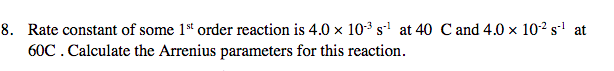# 8. Rate constant of some 1st order reaction is 4.0 x 103 s1 at 4060C. Calculate the Arrenius parameters for this reactionC and 4.0 x 102 s1 at

Question
1 viewshelp_outlineImage Transcriptionclose8. Rate constant of some 1st order reaction is 4.0 x 103 s1 at 40 60C. Calculate the Arrenius parameters for this reaction C and 4.0 x 102 s1 at fullscreen
check_circle

Step 1

Given:

Temperature T1 = 40 oC = 313 K.

Temperature T2 = 60 oC = 333 K.

Rate Constant at T1 (K1) = 4.0×10-3 s-1.

Rate Constant at T2 (K2) = 4.0×10-2 s-1.

Step 2

Arrhenius equation ...

### Want to see the full answer?

See Solution

#### Want to see this answer and more?

Solutions are written by subject experts who are available 24/7. Questions are typically answered within 1 hour.*

See Solution
*Response times may vary by subject and question.
Tagged in

### Chemical Kinetics